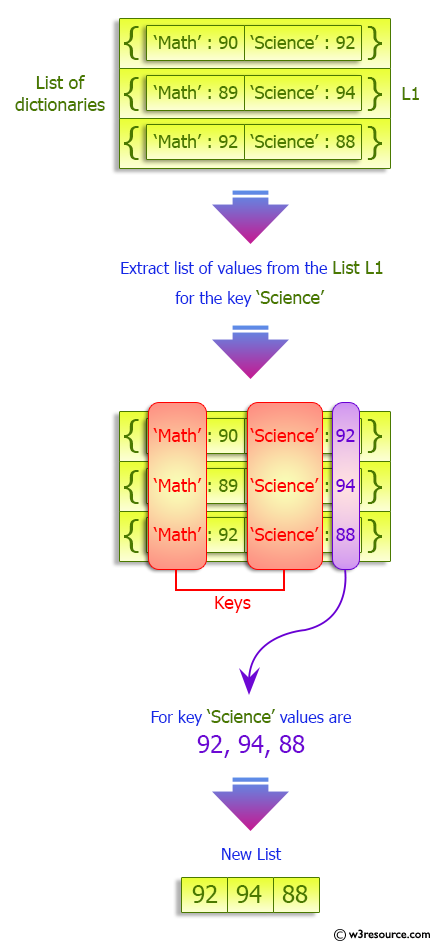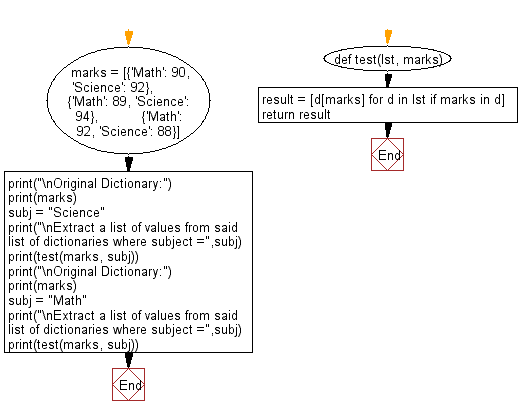﻿ Python: Extract a list of values from a given list of dictionaries - w3resource# Python: Extract a list of values from a given list of dictionaries

## Python dictionary: Exercise-52 with Solution

Write a Python program to extract a list of values from a given list of dictionaries.

Sample Solution:

Python Code:

``````def test(lst, marks):
result = [d[marks] for d in lst if marks in d]

return result

marks = [{'Math': 90, 'Science': 92},
{'Math': 89, 'Science': 94},
{'Math': 92, 'Science': 88}]

print("\nOriginal Dictionary:")
print(marks)
subj = "Science"
print("\nExtract a list of values from said list of dictionaries where subject =",subj)
print(test(marks, subj))

print("\nOriginal Dictionary:")
print(marks)
subj = "Math"
print("\nExtract a list of values from said list of dictionaries where subject =",subj)
print(test(marks, subj))
```
```

Sample Output:

```Original Dictionary:
[{'Math': 90, 'Science': 92}, {'Math': 89, 'Science': 94}, {'Math': 92, 'Science': 88}]

Extract a list of values from said list of dictionaries where subject = Science
[92, 94, 88]

Original Dictionary:
[{'Math': 90, 'Science': 92}, {'Math': 89, 'Science': 94}, {'Math': 92, 'Science': 88}]

Extract a list of values from said list of dictionaries where subject = Math
[90, 89, 92]
```

Pictorial Presentation:Flowchart:## Visualize Python code execution:

The following tool visualize what the computer is doing step-by-step as it executes the said program:

Python Code Editor:

Have another way to solve this solution? Contribute your code (and comments) through Disqus.

What is the difficulty level of this exercise?

Test your Programming skills with w3resource's quiz.

﻿

## Python: Tips of the Day

Invokes the provided function after ms milliseconds:

Example:

```from time import sleep

def tips_delay(fn, ms, *args):
sleep(ms / 1000)
return fn(*args)
print(tips_delay(
lambda x: print(x),
1000,
'w3r'
))
```

Output:

```w3r
None
```